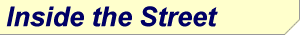Member Logon Home About Us Services Performance Subscription Investment Guide Wall Street Update Inside the Street Short-Term Long-Term Miscellaneous Affiliates Media Appearances IPO CenterTrading Curbs  If you spend much time following the market youve heard the term Standard Deviation mentioned.  Standard deviation has nothing to do with the normal mischievousness of a particular age group.  It is a statistical term, and a concept that can improve the success of your stock trading.  Its also the concept Bollinger Bands is based on.  In simplest of terms, standard deviation is a measure of how far a particular data point within a group is from the average.  You can also look at it as a measure of volatility or spread.   If all the data points of a group are near the average the standard deviation of the group would be low and the volatility would be low. The standard deviation is calculated by adding the squared variance from the average of all the data points.  You can calculate this with a pencil and piece of paper, but the good news is its a standard key on most any scientific calculator.  All you do is enter all the values of the group, i.e. the closing prices of a stock for the last ten days then press the standard deviation key and itll display the standard deviation for the values entered.  The displayed value will be one standard deviation from the mean.  Most of us have run into the concept of standard deviation and may not have realized it.  The bell curve used to grade students in high school or college is a representation of the concept of standard deviation.  A picture of the classic bell curve is an easy way to represent standard deviation.  A bell shape viewed from the side represents all the scores in the class if those scores follow a normal distribution.  A vertical line drawn from the highest point of the bell down to its base would represent the average score.  Most of the scores are grouped around the average score and these would be roughly within one standard deviation of the mean.  The scores to the extreme left and right of the bell would be within 2 or 3 standard deviations of the mean.   With the normal distribution of the bell curve the data points from one stand deviation below the mean to one standard deviation above the mean represent roughly 68 percent of the values in the group.  Two standard deviations (plus and minus) encompass 95 percent of the values in the group, and three standard deviations encompass 99 percent of the values in the group.  A teacher could use standard deviation to assign grades by simply figuring that any score greater than a value, i.e. 2.5 standard deviations above the mean receives an A, etc.  Applying the concept of standard deviation to investments gives the investor an idea of where a data point is relative to the mean and how volatile the markets are.  Bollinger Bands can be applied to any stock using most charting software.  Bollinger Bands overlay three lines on a stock chart.  The middle line is the moving average over the specified period, i.e. 14 days.  The top line is the value two standard deviations above the mean and the lower line is two standard deviations below the moving average.  As the market is more volatile the bands get broader and become more narrow as volatility is reduced.  How extreme a standard deviation value is depends on the length of the moving average in consideration.  Sometimes a market commentator will say that a particular market is 3 or 4 standard deviations above or below its given moving average.  A value 2 standard deviations from its mean is an extreme value.  If the current value of the index or stock is 3 or 4 standard deviations from its moving average then it is very extreme and often signifies a market top or bottom. This type of information can help an investor make money and the average investor can benefit from learning more about standard deviation and how it is used in stock market analysis. View our Past News Articles